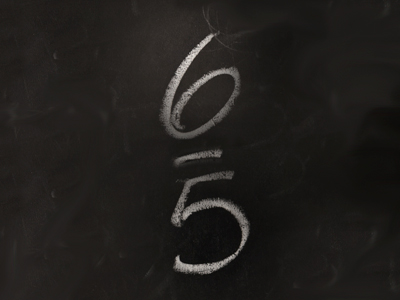65 is an improper fraction.

# Fractions (Year 5)

In KS2 Maths as children advance to Year Five their knowledge of fractions will increase. By now they should know the values of different fractions and will be introduced to numerators and denominators, proper and improper fractions and mixed numbers.

Fractions denote numbers that are less than a one. One way of writing these 'partial' numbers is by using a decimal point. These are called decimal numbers. An example would be 2.5. 2.5 as a fraction would be written as 212. 212 is a mixed number. Another way to write it would be 52 which is an improper fraction. Proper fractions have numerators which are lower than their denominators.

Want to teach your child to understand fractions? Take a look at our Help With Fractions blog where we give some really useful advice and tips.

1.
What type of fraction is 65?
Mixed fraction
Equivalent fraction
Improper fraction
Unsuitable fraction
An improper fraction has a top number more than the bottom number
2.
Which of these is a proper fraction?
912
76
43
129
A proper fraction has a top number less than the bottom number
3.
Which fraction is more than 14?
28
312
620
25100
520 is equivalent to 14 so 620 is more
4.
Which fraction is less than 12?
51100
712
59
715
2 x 7 = 14 so 715 is slightly less than half
5.
What would 64 be when converted to a mixed number?
412
112
114
214
A mixed number is where there is a mix of a whole number and a fraction
6.
Which fraction is 25100 equivalent to?
14
18
12
15
100 ÷ 25 = 4
7.
What would 314 be when converted to an improper fraction?
114
58
134
133
There are 44 in 1 one so 3 ones = 124
8.
Which fraction is one ninth?
18
19
16
112
19 is the same as 1 ÷ 9
9.
Which of these fractions is not equivalent to 13?
26
412
39
210
210 is the same as 15
10.
Which is the largest of these fractions?
39
912
35100
12
912 is larger because 9 is closer to 12 than 3 is to 9, 35 is to 100 and 1 is to 2